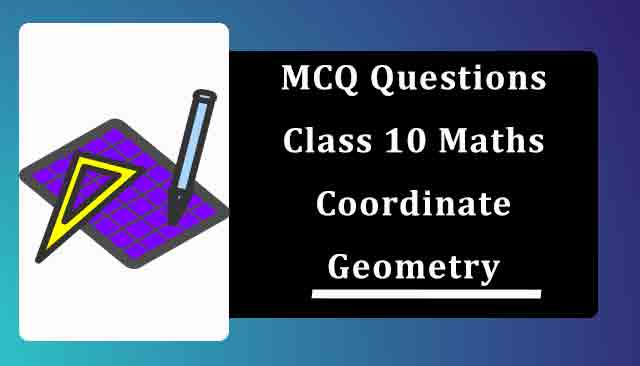MCQ Questions for Class 10 Maths Chapter 7 Coordinate Geometry with Answers

Below you will find NCERT MCQ Questions for Class 10 Maths Chapter 7 Coordinate Geometry with Answers which is very helpful during the preparation of examinations. These MCQ Online Test are one marks questions which will give you overview of the chapter in no time. One should try to understand Class 10 MCQ Questions as it is based on latest exam pattern released by CBSE.

Also, students can check NCERT Solutions for Class 10 Maths Chapter 7 for improving their marks and have good understanding of the chapter.Chapter 7 Coordinate Geometry MCQ Questions for Class 10 Maths with Answers

1. The distance of the point P (-6, 8) from the origin is
(a) 8
(b) 2√7
(c) 10
(d) 6
Solution (c) 10

2. The distance between the points (3,4) and (8,-6) is
(a) 2√5 units
(b) 3√5 units
(c) √5 units
(d) 5√5 units
Solution (d) 5√5 units

3. The length of the median through A of  ΔABC with vertices A(7, – 3), B(5, 3) and C(3, – 1) is
(a) 3 units
(b) 5 units
(c) 25 units
(d) 7 units
Solution (b) 5 units

4. If A is point on the x – axis whose abscissa is 5 and B is the point (1, – 3), then the distance AB is
(a) 5 units
(b) 25 units
(c) 9 units
(d) 8 units
Solution (a) 5 units

5. The area of a triangle whose vertices are A(2,7), B(3,-1) and C(-5,6) is​
(a) 28.5 sq. units
(b) 35 sq. units
(c) 30 sq. units
(d) 40 sq. units
Solution (a) 28.5 sq. units

6. The points (1,1), (-2, 7) and (3, -3) are
(a) vertices of an equilateral triangle
(b) collinear
(c) vertices of an isosceles triangle
(d) none of the above
Solution (b) collinear

7. If the points A(7,-2), B(5,1) and C(3,k) are collinear, then the value of K is:
(a) 4
(b) 2
(c) 8
(d) 6
Solution (a) 4

8. If the co – ordinates of a point are (3, – 7), then its ordinate is
(a) – 3
(b) – 7
(c) 3
(d) 7
Solution (b) – 7

9. The ordinate of a point is twice its abscissa. If its distance from the point (4,3) is v10, then the coordinates of the point are
(a) (1,2) or (3,6)
(b) (1,2) or (3,5)
(c) (2,1) or (3,6)
(d) (2,1) or (6,3)
Solution (a) (1,2) or (3,6)

10. If the points (8,1), (k,-4) and (2,-5) are collinear then the value of k is
(a) -3
(b) 3
(c) 9
(d) 0
Solution (b) 3

11. The vertices of a quadrilateral are (1, 7), (4, 2), (– 1, – 1) and (– 4, 4). The quadrilateral is a
(a) square
(b) rectangle
(c) parallelogram
(d) none of the above
Solution (a) square

12. The points which lies on the perpendicular bisector of the line segment joining the points A (-2, -5), B (2, 5) is
(a) (0, 0)
(b) (0, 2)
(c) (2, 0)
(d) (- 2, 0)
Solution (a) (0, 0)

13. The distance of the point P (2, 3) from the x-axis is
(a) 2
(b) 3
(c) 1
(d) 5
Solution (b) 3

14. If (3,0), (2,a), and (b,6) are the vertices of ABC whose centroid is (2,5), then the values of a and b are​
(a) a = 3, b = -9
(b) a = 0, b = 2
(c) a = 1, b = 9
(d) a = 9, b = 1
Solution (d) a = 9, b = 1

15. The points (a, b + c), (b, c + a) and (c, a + b) are​
(a) Non-collinear
(b) Collinear
(c) Concurrent
(d) All of the above
Solution (b) Collinear

16. The coordinates of the centre of a circle passing through (1, 2), (3, – 4) and (5, -6) is:
(a) (11, -2)
(b) (-2, 11)
(c) (11, 2)
(d) (2, 11)
Solution (c) (11, 2)

17. The distance between the points A (0, 7) and B (0, -3) is
(a) 4 units
(b) 10 units
(c) 7 units
(d) 3 units
Solution (b) 10 units

18. The base of an equilateral triangle ABC lies on the y – axis. The co – ordinates of the point C is (0, – 3). If origin is the midpoint of BC, then the co – ordinates of B are
(a) (0, 3)
(b) (–3, 0)
(c) (0, – 3)
(d) (3, 0)
Solution (a) (0, 3)

19. The distance between the points (a, a) and (−√3a,√3a) is
(a) 3√2a units
(b) 2√2a units
(c) 2√2 units
(d) 2 units
Solution (b) 2√2a units

20. The distance between the point P(1, 4) and Q(4,0) is
(a) 4
(b) 5
(c) 6
(d) 3√3
Solution (b) 5

21. The area of triangle ABC with A(3,2), B(11,8) and C(8,12) in square units is​
(a) 20
(b) 25
(c) 35
(d) 30
Solution (b) 25